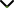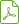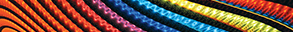# Recent Theory and Applications on Numerical Algorithms and Special Functions

Publishing date
06 Feb 2015
Status
Published
19 Sep 2014

Guest Editors

1King Abdulaziz University, Jeddah, Saudi Arabia

2University of Central Florida, Orlando, USA

3Cankaya University, Ankara, Turkey

4Neijiang Normal University, Neijiang, China

# Recent Theory and Applications on Numerical Algorithms and Special Functions

## DescriptionIn recent years, there has been extensive research activity on both the theory and application of efficient numerical methods for approximating the solutions of differential equations. Numerical algorithms and special functions have been a major thrust of this research and have numerous applications in science and engineering. Special functions play a tremendous role in mathematical analysis and in the development of new numerical techniques. In particular, they allow a broad refinement of spectral methods for solving several types of differential equations in both finite and infinite domains. This is useful, in that there is a strong need for efficient and easy-to-use scalable techniques for the numerical solution of large scale problems.

This special issue focuses on the analysis of numerical methods and special functions, while also addressing the practical nature of the two fields. In addition to the strong focus in computational mathematics, scientific computing, and theoretical physics, a special emphasis will be given to orthogonal polynomials, the theory of numerical methods for solution of differential equations, and applications of these fields.

Potential topics include, but are not limited to:

• Numerical methods for integral equations
• Fractional differential equations
• Dynamics of nonlinear differential equations
• Functional differential equations on various time scales
• Impulsive differential and integral equations
• Fixed-point theory relevant to numerical methods
• Numerical solution of partial differential equations and relevant applications
• Analytical methods for nonlinear differential equations
• Numerical optimization
• Scientific computing
• Applications of the above in science and engineeringDownload Special IssueJournal metrics
See full report
Acceptance rate13%
Submission to final decision68 days
Acceptance to publication21 days
CiteScore1.500
Journal Citation Indicator-
Impact Factor-Author guidelinesEditorial boardDatabases and indexing

Article of the Year Award: Outstanding research contributions of 2021, as selected by our Chief Editors. Read the winning articles.